StatLect

# Wald test

The Wald test is a test of hypothesis usually performed on parameters that have been estimated by maximum likelihood (ML).## The null hypothesis

We assume that an unknown-dimensional parameter vectorhas been estimated by ML.

We want to test the null hypothesis thatequations (possibly nonlinear) are satisfied:whereis a vector valued function, with.

The lecture on hypothesis testing in maximum likelihood framework explains that the most common null hypotheses can be written in this form.

## Assumptions

Let the parameter space be.

We assume that the following technical conditions are satisfied:

• for each, the entries ofare continuously differentiable with respect to all the entries of;

• thematrix of the partial derivatives of the entries ofwith respect to the entries of, called the Jacobian ofand denoted by, has rank.

## The ML estimator

Letbe the estimate of theparameterobtained by maximizing the log-likelihood over the whole parameter space:whereis the likelihood function andis the sample.

We assume that the sample and the likelihood function satisfy some set of conditions that are sufficient to guarantee the consistency and asymptotic normality of(see the lecture on maximum likelihood for a set of such conditions).

## The Wald statistic

Here is the formula for the test statistic used in the Wald test: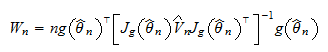whereis the sample size, andis a consistent estimate of the asymptotic covariance matrix of(see Covariance matrix of the MLE).

## The asymptotic distribution of the test statistic

Asymptotically, the test statistic has a Chi-square distribution.

Proposition Under the null hypothesis that, the Wald statisticconverges in distribution to a Chi-square distribution withdegrees of freedom.

Proof

We have assumed thatis consistent and asymptotically normal, which implies thatconverges in distribution to a multivariate normal random variable with meanand asymptotic covariance matrix, that is,Now, by the delta method, we have thatBut, so that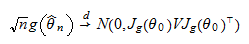We have assumed thatandare consistent estimators, that is,wheredenotes convergence in probability. Therefore, by the continuous mapping theorem, we have that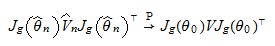Thus we can write the Wald statistic as a sequence of quadratic formswhereconverges in distribution to a normal random vectorwith mean zero, andconverges in probability to. By a standard result (see Exercise 2 in the lecture on Slutsky's theorem), such a sequence of quadratic forms converges in distribution to a Chi-square random variable with a number of degrees of freedom equal to.

## The test

In the Wald test, the null hypothesis is rejected ifwhereis a pre-determined critical value.

The size of the test can be approximated by its asymptotic value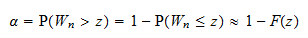whereis the distribution function of a Chi-square random variable withdegrees of freedom.

The critical valueis chosen so as to achieve a pre-determined size, as follows:## Example

This example shows how to use the Wald test to test a simple linear restriction.

Let the parameter space be the set of all-dimensional vectors:### The estimates

Suppose that we have obtained the following estimates of the parameter and of the asymptotic covariance matrix:whereis the sample size.

### The null hypothesis

We want to test the restrictionwhereanddenote the first and second entries of.

Then, the functionis a functiondefined by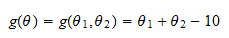In this case,.

### The Jacobian

The Jacobian ofis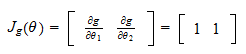which has rank.

Note also that it does not depend on.

### The test statistic

We haveWe can substitute these values in the formula for the Wald statistic: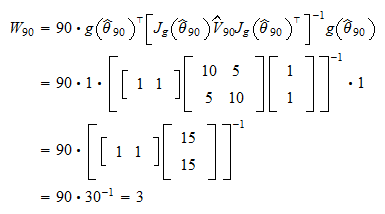Our test statistic has a Chi-square distribution withdegrees of freedom.

### The critical value

Suppose that we want our test to have size.

Then, our critical valueis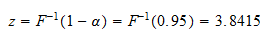whereis the distribution function of a Chi-square random variable withdegree of freedom.

The value ofcan be calculated with any statistical software (for, example, in MATLAB with the command `chi2inv(0.95,1)`).

### The decision

Therefore, the test statistic does not exceed the critical valueand we do not reject the null hypothesis.